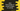# Java program to find the number of vowels and digits in a String## Find number of vowels and digits in a String using Java:

In this tutorial, we will learn how to calculate the total number of vowels and digits in a String .

1. We are using Scanner class to get the input from the user.
2. Then using a for loop, we will check each character of that string.
3. Using a if condition, we will check if the character is equal to any vowel.
4. Both lower case and upper case ‘AEIOU’ and ‘aeiou’ should be considered while checking.
5. If the character is vowel, increment the counter.
6. If the character is not vowel, check if it is a digit using Character.isDigit() method.
7. If it is a digit, increment the counter for digit.
8. After the loop is completed, print both counters.

### Program :

``````import java.util.Scanner;

public class Main {

public static void main(String[] args) {
String inputStr;

int v = 0;
int n = 0;

Scanner scanner = new Scanner(System.in);

inputStr = scanner.nextLine();

for (int i = 0; i < inputStr.length(); i++) {
Character c = inputStr.charAt(i);
if (c == 'A' || c == 'E' || c == 'I' || c == 'O' || c == 'U' || c == 'a' || c == 'e' || c == 'i' || c ==
'o' || c == 'u') {
v++;
} else if (Character.isDigit(c)) {
n++;
}
}

System.out.println("No of vowels "+v);
System.out.println("No of numbers "+n);

}

}``````

Instead of checking each vowel characters, we can use public int indexOf(int ch) method of the String class.It takes a character as parameter and returns the index within the string of the first occurrence of the character. If character is not found, it returns -1. So, -1 means the character is not vowel if we call this method for string ‘AEIOUaeiou’, isn’t it ? Let’s modify the above program :

``````import java.util.Scanner;

public class Main {

public static void main(String[] args) {
String inputStr;

int v = 0;
int n = 0;

Scanner scanner = new Scanner(System.in);

inputStr = scanner.nextLine();

for (int i=0 ; i<inputStr.length(); i++){
Character c = inputStr.charAt(i);
if("AEIOUaeiou".indexOf(c) != -1){
v ++;
}else if(Character.isDigit(c)){
n++;
}
}

System.out.println("No of vowels "+v);
System.out.println("No of numbers "+n);
}

}``````